#### You may also like### Homes

Six new homes are being built! They can be detached, semi-detached or terraced houses. How many different combinations of these can you find?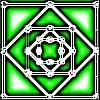### Number Squares

Start with four numbers at the corners of a square and put the total of two corners in the middle of that side. Keep going... Can you estimate what the size of the last four numbers will be?### I'm Eight

Find a great variety of ways of asking questions which make 8.

# Routes 1 and 5

##### Age 5 to 7Challenge Level

Fiona from Tattingstone School tackled this very clearly: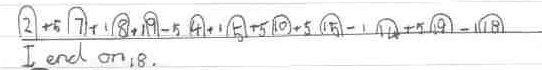She found another way of starting and ending on these numbers: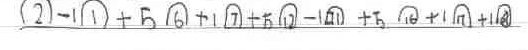Fiona then explains: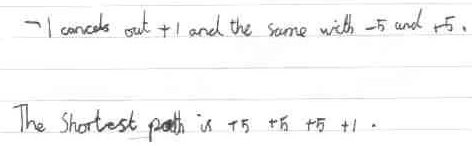Omar from the Modern English School, Cairo drew out a few different routes which also start at $2$ and end at $18$: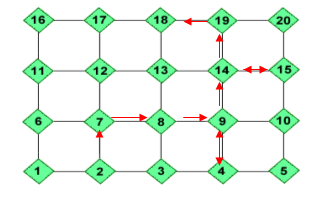I like the way you've shown the 'optional extras' with double-headed arrows, Omar Abdel also from the Modern English School found another route:

$2+1+1+5-5+1+5+5-1+5-1$

Elliot, Richard and Christopher from Moorfield Junior School agreed with Fiona but also found another equally short route: $+1,+5,+5,+5$.

Molly and Callum from Bradon Forest School sent us a detailed response:

The last number in the sequence is $18$, and another Sequence is $2(+5)7(+5)12(+5)17(+1)18$.
But the last one is the hardest. Still using the example $2$-$18$ above you need to subtract the biggest number from the smallest one ($18-2=16$)
The number you are left with is the number that all the steps shold add up to, or 'special number' ($5+5+5+1=16$) or ($5+1+1-5+1+5+5-1+5-1=16$) To find out the number of steps and what they are add keep adding together $5$'s until you reach the number closest to the special number ($3\times5=15$ is closer to $16$ than $4\times5=20$). Then continue adding or subtracting $1$'s until you get the special number ($15+(1\times1)=16$). This might be a bit clearer;
$3\times5 = 15$
$1\times1 = 01$ = $16$ --special number in four steps
You can try that on any sequence and it'll still work!!!

Luke from Witton Middle School noticed something important:

For the first part of the challenge, I worked out that the number you ended on was $1$8.
So for the second part of the challenge, I did:
$2 +1 (3) +5 (8) -1 (7) +5 (12) -1 (11) +5 (16) +1 (17) -5 (12) +1 (13) +5 =18$
Then I used cancelling down (for example, $+1$ and $-1$ can be cancelled out because whatever number is the input, the output will be the same as the input. e.g. $32 +1 -1 =32$).
To end up with the shortest route - $2 +5 +5 +5 +1 =18$.
You could add in a different order but the answer would be the same.

Well done, Luke, you're right that the order of the operations is not important - you would still get to $18$.